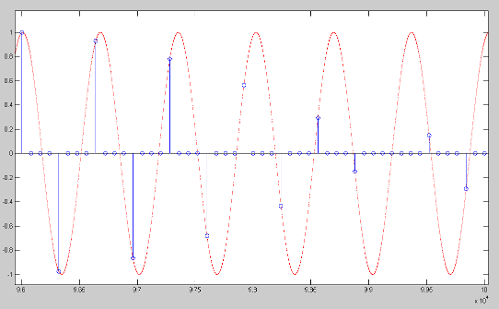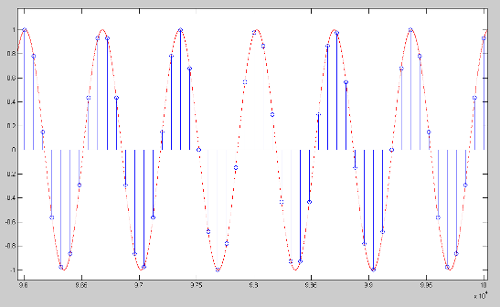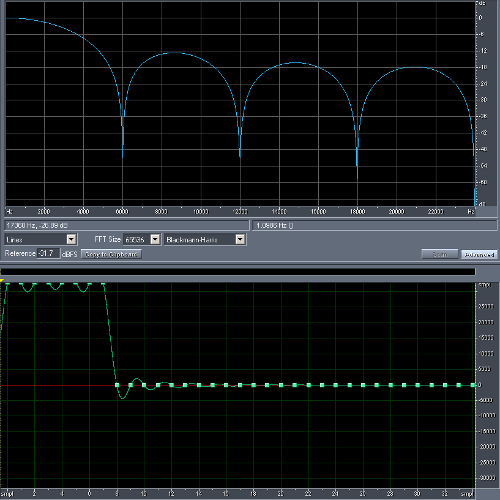# Resampling explained

Discussion in 'Sound Science' started by xnor, Dec 10, 2012.

1. Hello,

since I've noticed a couple of times that people confuse upsampling, resampling etc. I thought I should try to explain these terms.

Before I can explain resampling though I have to explain upsampling, interpolation, downsampling and decimation.

Upsampling
is the simple process of inserting zeros between the original samples to increase the sampling rate (fs).

For example, adding one zero between each sample doubles the sampling rate but also introduces undesired spectral images above the original Nyquist frequency (fs / 2).

Interpolation
is the process of upsampling followed by filtering to remove the undesired spectral images.

The filter is a low-pass filter that ideally completely eliminates all frequencies above the original Nyquist frequency while passing those below (the original signal) unchanged.

The interpolation factor is usually symbolized by "L".
L = target fs / source fs.

Downsampling
is the simple process of throwing away samples to reduce the sampling rate.

For example, throwing away every other sample halves the sampling rate.

Decimation
is the process of filtering (to avoid aliasing) followed by downsampling.

The decimation factor is usually symbolized by "M".
M = source fs / target fs.

Resampling (sample rate conversion)
is the combination of interpolation and decimation to change the sampling rate by a rational factor.

For example, if we want to resample 44100 Hz (CD audio) to 96000 Hz we have a resampling factor of: 2.17687...
Though, to be able to interpolate and decimate we need integer factors.

So if we use:

L = 320
M = 147

then we first interpolate: 44100 Hz * 320 = 14112000 Hz
and then decimate: 14112000 Hz / 147 = 96000 Hz

of course only one low-pass filter is necessary, to remove undesired spectral images above the lower fs / 2. In this case: 22050 Hz.

Efficient implementations will only calculate the sample values that appear on the output.

Any questions?

anetode and ultrabike like this.
2. So essentially re-sampling is a super set of up/down sampling.

3. Yeah, that plus filtering.

4. I have recorded sound on my I phone camera set on Video mode. The sound was a high sharp noise. But when I play back the recording I here audio voices that sound like chip and dale. Any idea on how I can splice it up?

Here's an example of the interpolation process.

The image shows a 21 kHz sine wave, sampled at 44.1 kHz with 3 zeros inserted between each original sample (= upsampling by 4x).If we interpolate, i.e. filter out the spectral images introduced by upsampling, we get this:As you can see, all these additional (redundant) samples can be reconstructed simply by interpolation.

6. In the first image, why does it seem like there's some aliasing?

I guess a simple way to upsample would be to first do 2x sampling, where a new sample is just an average of adjacent samples. Then to make it 4x, introduce one more sample each between the newly added sample, the previous sample, and next sample. Followed by some low pass filtering.

7. Quote:
There is no aliasing since the signal (a single 21 kHz sine wave) is band-limited to below fs/2 (= 22.05 kHz).

A full 21 kHz sine wave cycle has 44.1/21 = 2.1 samples and that's what makes it look "odd". There's no even number of samples per cycle so they will shift 0.1 samples per cycle. Had I chosen 14.7 kHz instead of 21 kHz you'd see exactly 44.1/14.7 = 3 samples per cycle.
I chose 21 kHz intentionally to show that thinking in "straight lines" does not work.

Quote:
If you look at the non-zero samples in the first image and add average samples in between you will see that the result will be anything but a clean 21 kHz sine wave.

To get high sound quality from a resampler you need a proper filter, typically a windowed sinc filter. Linear interpolation (= averages) only works well if you have oversampled the signal, i.e. sampled the signal with a much higher sampling rate than needed.
For a >96 dB SNR with linear interpolation you need a sampling rate of 5644.8 kHz (= 128 * 44.1 kHz)!!!

8. Quote:

Yeah, its not exactly aliasing, more like an envelope curve.

9. Quote:

To up-sample from 48 KHz to 96 KHz, wouldn't you simply repeat each sample, rather than insert a zero?

--Ethan

10. The (amplitude) envelope of the signal would actually be a straight line at +1.0 and can be computed using the Hilbert transform, but that's beyond this thread's topic.

The instantaneous value of the continuous signal (the red sine wave) is taken at fixed intervals, whatever the value is. You can check this on your own by generating the same signal and taking a look at it in an audio editor.
Besides the samples, good editors will show a sine wave. Others will connect the samples with straight lines (= linear interpolation) or display a "staircase" line (= zero-order hold).
Both of these techniques are used in some resamplers and even DACs. But the highest quality is achieved with zero-stuffing and a proper low-pass filter.

Quote:
This is zero-order hold. This technique still creates undesired spectral images but it also creates distortion in the passband. (Remember from the first post that an ideal low-pass doesn't "touch" the original signal.)
Of course you can counteract this passband distortion with the filter, but it's usually more efficient and cleaner to just use zero-stuffing.

A multiplication with a zero-valued sample always equals zero. Now if you know you have L-1 zeros between each original sample you can use that information to speed up the resampling process a lot, by skipping those pointless multiplications.

11. Quote:
Do you have any non-math references that explain the difference between duplicating samples and "zero stuffing" I can read?

12. Repeating samples is basically like inserting zeros first (which has a flat response for both the original signal and the images created above the Nyquist frequency), and then convolving the result with an impulse response that consists of L samples at the full scale level. That is a simple lowpass/comb filter, as shown by the image below with L=8:13. ^ Right.

The most simple explanation I could think of is this:

Create a file with a sampling rate of 44100 Hz with a single sample with the value 1.0 (= impulse).
Take a look at the frequency response: flat from DC to 22050 Hz (= fs/2).

Now lets double the sampling rate (if you try this in an audio editor you have to "interpret" the sample rate as 88200 Hz after the changes):

a) add a zero, the FR still is the same from DC to 22050 Hz, but we now also have a spectral image from 22050 Hz to 44100 Hz (= the new fs/2).

As stv014 wrote, we now have a comb filter. The FR has a notch at 44100 Hz. This causes some roll-off below the old fs/2.

14. Bear with me here guys.I created a 44.1 KHz file in Sound Forge containing a 1 KHz sine wave at - 6dBFS. Zooming way in showed 44 individual samples within a single cycle. Then I told SF to re-sample to 88.2 and zoomed in again. Then there were 88 samples in one cycle. I didn't really expect to see zero-level samples in between the original samples! What am I missing?

Edit: I understand that the zero-level samples don't appear in the data because they're higher in frequency than audio and are filtered out. So the better question is, why doesn't inserting zeroes reduce the level to half?

--Ethan

15. I'm not familiar with most audio editors, but I presume that what is being done is sample rate conversion (which is what people want), not just the upsampling itself. They're doing the upsampling + interpolation filter to get to the other sampling rate, meaning what you see is the correct waveform at the higher sampling rate.

My DSP chops are minimal at best, and it's been a while so my terminology may be off, but I think the confusion comes from "upsampling" being used loosely in many settings. Properly, or at least as it's defined here, upsampling just refers to adding the zeros in between samples, not the whole process. If you convert from 44.1 kHz to 88.2 kHz, that is sample rate conversion (in this case, it only requires upsampling followed by the low-pass interpolation filter). To convert from 88.2 kHz to 44.1 kHz, you need to low-pass filter and then downsample. To convert by a non-integer factor, you need to upsample, low-pass filter, and then downsample.

That said, mathematically and practically, there is more than one way to do the same thing. By changing the filter, you can switch the order of filtering and downsampling or upsampling.

Anyhow, the interpolation filter needs to increase the level by a factor of L in the passband to maintain the same level; good catch. That's part of the conversion process behind the scenes.